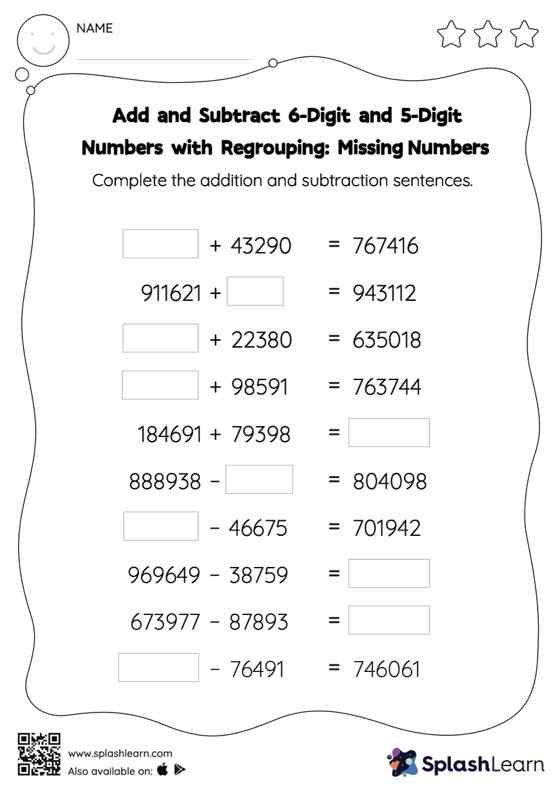# Add and Subtract 6-Digit and 5-Digit Numbers with Regrouping: Missing Numbers Worksheet

Home > Add and Subtract 6-Digit and 5-Digit Numbers with Regrouping: Missing NumbersTo find the missing number on this worksheet, students can utilize the connection between addition and subtraction. In add and subtract 6-digit and 5-digit numbers with regrouping worksheet, they must additionally regroup numbers to reach the solution. This worksheet is about practicing with the horizontal format in which numbers are written side by side. To develop flexibility with numbers and operations, students need to have enough practice in this format and not just rely on the vertical/column method.